Published: 15 August 2014

# Vibration suppression using fractional-order disturbance observer based adaptive grey predictive controller

Jianshan Lu1
Weidong Xie2
Hongbo Zhou3
Aijun Zhang4
1, 2, 3Vehicle Engineering Research Institute, Zhejiang University of Technology, Hangzhou, 310014, China
4Key Laboratory of Intelligent Perception and Systems for High-Dimensional Information, Ministry of Education, Nanjing University of Science and Technology, Nanjing, 210094, China
Corresponding Author:
Jianshan Lu
Views 44

#### Abstract

A novel control strategy is proposed for vibration suppression using an integration of a fractional-order disturbance observer (FDOB) and an adaptive grey predictive controller (AGPC). AGPC is utilized to realize outer loop control for better transient performance by predicting system outputs ahead with metabolic GM(1,1) model, and an adaptive step switching module is adopted for the grey predictor in AGPC. FDOB is used to obtain disturbance estimate and generate compensation signal, and as the order of $Q$-filter is expanded to real-number domain, FDOB has a wider range to select a suitable tradeoff between robustness and vibration suppression. For implementation of the fractional order $Q$-filter, broken-line approximation method is introduced. The proposed control strategy is simple in control-law derivation, and its effectiveness is validated by numerical simulations.

## 1. Introduction

Grey System Theory, pioneered by Deng (1982), can effectively deal with uncertain problems with less data and poor information. And on the basis of grey prediction models, grey predictive controller (GPC) which has been successfully applied in many control fields, can be employed to avoid future adverse events from happening. In [1-3], PID based grey predictive controllers were used to control the sliding inverted pendulum, dynamic tension during cold rolling, vegetable grasping system, respectively. Wang et al. presented a grey fuzzy PI controller for packing pressure control during the injection process in . Chung et al. also adopted a grey prediction fuzzy controller to provide pH control in the food industry . In , Erdal and Okyay took a non-linear liquid level system as a test bed to examine the grey fuzzy PID controller, and the results indicate that grey predictive controller has the ability to control the non-linear system accurately with little overshoot, with no steady-state error. However, in practice, a physical control system will not be the exactly same as a mathematical model no matter how the model is obtained . And vibration occurs in most machines, structures, and dynamic systems, which causes noise, reduces accuracy and reliability of equipments [8, 9]. Thus, to improve disturbance rejection characteristics and command following characteristics for grey predictive control systems, disturbance observer (DOB) is introduced in this study. At present, DOB-based control techniques for linear and nonlinear systems have been developed and used in a lot of control fields, e.g., hard disk drive systems , flight simulator , micro machine tools , inertially stabilized platforms , space manipulator, and general systems . When applying DOB, there is a tradeoff between the phase margin loss and the strength of the low frequency vibration suppression . But as the order of $Q$-filter in DOB just can be chosen in integer domain, the ideal tradeoff between stability margin loss and vibration suppression can hardly be reached. Recently, applications of fractional calculus theory in practical control field have increased significantly , and a fractional-order disturbance observer (FDOB) based on the fractional order $Q$-filter is proposed, too . The FDOB can adjust the tradeoff between stability margin loss and vibration suppression simultaneously and continuously. Ma and Li used fractional-order disturbance observers in speed control of torsional systems respectively, and experimental results show that changing the $Q$-filter’s order fractionally can give a more effective way to adjust control system’s frequency and time responses than just turning it among integer orders [8, 15].

Therefore, in this study, a novel control strategy would be proposed using an integration of a fractional-order disturbance observer and an adaptive grey predictive controller (AGPC). FDOB is used to estimate the disturbance for the feedforward compensation, and AGPC is utilized to realize outer loop control to achieve better transient performance. The remainder of this paper is organized as follows. In Section 2, the fundamental structures of GPC and DOB are described. Section 3 introduces the design of the newly proposed controller detailedly, including the designs of adaptive switching mechanism and fractional order $Q$-filter. Then in Section 4, some numerical simulations are carried out to demonstrate the effectiveness of the novel control strategy. Finally concluding remarks are presented in Section 5.

## 2. Preliminaries

### 2.1. Grey predictive control structure

Grey predictive control strategy is designed based on the control decision made on the basis of system future behavioral tendency, and the grey predicted outputs could always provide us some useful information for better control of the system before the system behavior runs into bad situations . The control structure is depicted in Fig. 1.

Fig. 1Grey predictive control structure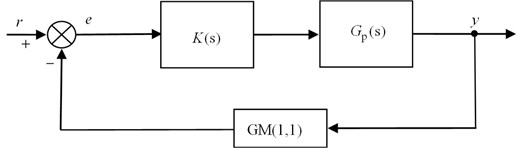In Fig. 1, ${G}_{p}\left(s\right)$ is plant transfer function, $K\left(s\right)$ is the regulator, and it can take PID or other control modes, GM(1,1) module is the grey prediction module. Signals $r$, $y$, $e$ denote the reference input, system output and tracking error respectively. Let $\mathbf{Y}=\left\{y\left(k\right),\text{\hspace{0.17em}}\text{}k=1,\text{\hspace{0.17em}}2,\dots ,\text{\hspace{0.17em}}n\right\}$ stand for a sample of the output components with $y\left(k\right)$ being the output at time $k$. And via translational processing, we can obtain a positive sequence ${\mathbf{Y}}^{\left(0\right)}=\left\{{y}^{\left(0\right)}\left(k\right),\text{\hspace{0.17em}}\text{}k=1,\text{\hspace{0.17em}}2,\dots ,\text{\hspace{0.17em}}n\right\}$. Then GM(1,1) model could be constructed with the positive sequence ${\mathbf{Y}}^{\left(0\right)}$ by the following basic steps .

First, apply a preliminary transformation called accumulated generating operation (AGO) to reduce the noise of ${\mathbf{Y}}^{\left(0\right)}$ and to get a new monotonically increasing data sequence ${\mathbf{Y}}^{\left(1\right)}=\left\{{y}^{\left(1\right)}\left(k\right)|{y}^{\left(1\right)}\left(k\right)=\sum _{i=1}^{k}{y}^{\left(0\right)}\left(i\right),k=1,\text{\hspace{0.17em}}2,\dots ,\text{\hspace{0.17em}}n\right\}$. Then the grey differential equation can be formed as follows:

1
${y}^{\left(0\right)}\left(k\right)+a{z}^{\left(1\right)}\left(k\right)=b,k=2,\text{\hspace{0.17em}}3,\dots ,\text{\hspace{0.17em}}n,$

where ${z}^{\left(1\right)}\left(k\right)=0.5\left[{y}^{\left(1\right)}\left(k\right)+{y}^{\left(1\right)}\left(k-1\right)\right]$, $a$ is developing coefficient and $b$ is the grey input.

Adopt least square method to estimate the model parameters ${\left[ab\right]}^{T}$:

2
${\left[a\text{}b\right]}^{\text{T}}={\left({\mathbf{B}}^{T}\mathbf{B}\right)}^{-1}{\mathbf{B}}^{T}{\mathbf{Y}}_{N},$

where $\mathbf{B}$, ${\mathbf{Y}}_{N}$ are the data matrix and data vector respectively:

$\mathbf{B}=\left[\begin{array}{cc}-{z}^{\left(1\right)}\left(2\right)& 1\\ -{z}^{\left(1\right)}\left(3\right)& 1\\ ⋮& ⋮\\ -{z}^{\left(1\right)}\left(n\right)& 1\end{array}\right],{\mathbf{Y}}_{N}=\left[\begin{array}{c}{y}^{\left(0\right)}\left(2\right)\\ {y}^{\left(0\right)}\left(3\right)\\ ⋮\\ {y}^{\left(0\right)}\left(n\right)\end{array}\right].$

White-formed ordinary differential equation of grey model GM(1,1) is:

3
$\frac{\text{\hspace{0.17em}}{dy}^{\left(1\right)}}{dt}+a{y}^{\left(1\right)}=b.$

Solve Eq. (3) with the obtained model parameters ${\left[ab\right]}^{T}$ and apply the inverse AGO (IAGO) to figure out predictive values:

4
${\stackrel{^}{y}}^{\left(0\right)}\left(n+\xi \right)={\stackrel{^}{y}}^{\left(1\right)}\left(n+\xi \right)-{\stackrel{^}{y}}^{\left(1\right)}\left(n+\xi -1\right)=\left(1-{e}^{a}\right)\left({y}^{\left(0\right)}\left(1\right)-\frac{b}{a}\right){e}^{-a\left(n+\xi -1\right)},$

where $\xi$ is prediction step.

Finally, via inverse translational processing, we can obtain the predictive system output $\stackrel{^}{y}\left(n+\xi \right)$ which will be sent to the controller. In a grey predictive control system, predictions are often done using metabolic models. Thus, the parameters of the prediction device vary with time, and this would guarantee a strong adaptability of the system.

### 2.2. Disturbance observer

As is well-known, the performance of the closed-loop system will degrade in the presence of external disturbances, and in order to enhance the disturbance rejection performance of system, the DOB is introduced . DOB is located inside the control closed-loop, and it can further reduce the influence of vibration and model perturbation on the basis of closed-loop regulation. The basic idea of DOB is to use a nominal model of the plant to estimate the disturbance caused by outer interference torque and parameter variation, and an equivalent compensation action is generated from the estimate . The architecture of DOB is depicted in Fig. 2, where ${G}_{n}^{-1}\left(s\right)$ is the inverse of nominal model, $Q\left(s\right)$ is a low-pass filter, and $d$ is the equivalent disturbance. The relative order of ${G}_{n}\left(s\right)$ is usually not equal to zero, and this leads to that the inverse ${G}_{n}^{-1}\left(s\right)$ cannot be realized physically. Thus $Q$-filter is introduced to solve the problem in DOB.

Fig. 2Block diagram of DOB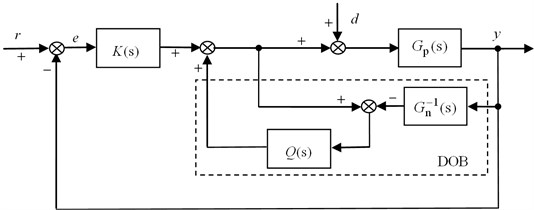In Fig. 2, system output $y$ can be calculated by:

5
$y=\frac{K\left(s\right){G}_{p}\left(s\right){G}_{n}\left(s\right)}{\left(1+K\left(s\right){G}_{p}\left(s\right)\right){G}_{n}\left(s\right)+\left({G}_{p}\left(s\right)-{G}_{n}\left(s\right)\right)Q\left(s\right)}\cdot r$
$+\frac{{G}_{p}\left(s\right){G}_{n}\left(s\right)\left(1-Q\left(s\right)\right)}{\left(1+K\left(s\right){G}_{p}\left(s\right)\right){G}_{n}\left(s\right)+\left({G}_{p}\left(s\right)-{G}_{n}\left(s\right)\right)Q\left(s\right)}\cdot d.$

Assumption 1. The closed-loop system is stabilized by $K\left(s\right)$, and the disturbance $d$ has a bounded steady-state value, i.e., the value satisfies the equation $\underset{t\to \infty }{\mathrm{l}\mathrm{i}\mathrm{m}}d\left(t\right)=\underset{s\to 0}{\mathrm{l}\mathrm{i}\mathrm{m}}sD\left(\text{s}\right)<\infty$ .

Assumption 2. The $Q$-filter in DOB is an ideal low-pass filter with a cutoff frequency ${w}_{q}$, it means that when $w<{w}_{\text{q}}$, $Q\left(\text{s}\right)\approx \text{1}$, and when $w>{w}_{\text{q}}$, $Q\left(\text{s}\right)\approx \text{0}$.

Now, with the above two assumptions, Eq. (5) can be approximated as:

6
$y\approx \frac{K\left(s\right){G}_{n}\left(s\right)}{1+K\left(s\right){G}_{n}\left(s\right)}\cdot r.$

From Eqs. (5) and (6) we can find that, DOB can effectively suppress the external disturbance, and make system characteristic approximately equal to nominal model.

## 3. Fractional-order DOB based adaptive grey predictive controller

Considering the advantages of the two mentioned controllers, we propose a novel control strategy using an integration of a disturbance observer and a grey predictive controller. Since the prediction step size decides the predictive value and finally affects the control performance, an adaptive step switching module is adopted to regulate the appropriate prediction step size of the grey predictor. And in order to reach an ideal tradeoff between stability margin loss and vibration suppression, the fractional order $Q$-filter is introduced. Therefore, we can obtain the architecture of fractional-order DOB based adaptive grey predictive controller (FDOB-AGPC) as depicted in Fig. 3, where AS module is an adaptive step switching module.

Fig. 3Block diagram of FDOB-AGPC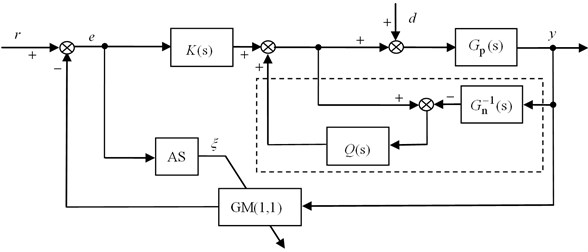### 3.1. Adaptive step switching module

In general, when system tracking error $e$ is large, the controller should transmit a big control signal to speed up the system response and result in a shorter settling time. And when the system error is small, the controller should generate a smaller control signal to prevent the system overshoot. Thus, for obtaining an excellent system control performance, we use the following switching mechanism in AGPC to determine prediction step size which would affect the generated control signal :

7
$\xi =\left\{\begin{array}{ll}{\xi }_{1},& e>{e}_{l},\\ {\xi }_{2},& {e}_{s}\le e\le {e}_{l},\\ {\xi }_{3},& e<{e}_{s},\end{array}\right\{\xi }_{3}>{\xi }_{2}>0>{\xi }_{1},$

where ${e}_{s}$ and ${e}_{l}$ are the switching values, ${\xi }_{1}$, ${\xi }_{2}$, and ${\xi }_{3}$ are the step sizes for the large error, the middle error and the small error respectively. The negative-step can increase the upward momentum of the output curve for shortening the settling time, and appropriate positive-steps can be used to prevent the overshooting.

### 3.2. Fractional order $\mathbit{Q}$-filter design

The design of $Q$-filter is an important step when applying DOB, and $Q$-filter characterizes the overall performance of the designed DOB. If given the required cutoff frequency of $Q$-filter, it turns out that the relative degree is the major turning knob for the tradeoff between stability margin loss and vibration suppression . Conventional DOB chooses the relative degree just in integer domain, and in order to further improve vibration suppression performance while keep enough stability margin, the filter order can be expanded to real-number domain. The fractional order version of $Q$-filter is:

8
$Q\left(s\right)=\frac{1}{{\left(\tau s+1\right)}^{\alpha }},\alpha \in R,$

where $\tau$ is the filter time constant and $\alpha$ is the relative degree of $Q$-filter. For implementation of the fractional order $Q$-filter, proper approximation by finite difference equation is needed. In this study, the broken-line approximation method is introduced to approximate $1/{\left(\tau \text{s}+1\right)}^{\alpha }$ in frequency range $\left[{w}_{b}, {w}_{h}\right]$, where ${w}_{\text{b}}=1/\tau$, ${w}_{\text{h}}$ is taken as 104 to give an enough frequency range . Let:

9
$G\left(s\right)={\left(\frac{1+s/{\omega }_{h}}{1+s/{\omega }_{b}}\right)}^{\alpha }.$

Eq. (9) can be approximated with the approach of cascading rational functions, and there is:

10
${G}_{N}\left(s\right)=\prod _{i=0}^{N-1}\frac{1+s/{\omega }_{i}^{\text{'}}}{1+s/{\omega }_{i}},G\left(s\right)=\underset{N\to \infty }{lim}{G}_{N}\left(s\right),$

where:

${\omega }_{i}={\omega }_{b}{\left(\frac{{\omega }_{h}}{{\omega }_{b}}\right)}^{\left(i+\frac{1}{2}-\frac{\alpha }{2}\right)/N},{\omega }_{i}^{\text{'}}={\omega }_{b}{\left(\frac{{\omega }_{h}}{{\omega }_{b}}\right)}^{\left(i+\frac{1}{2}+\frac{\alpha }{2}\right)/N}.$

Fig. 4Bode plots of ideal case and its approximations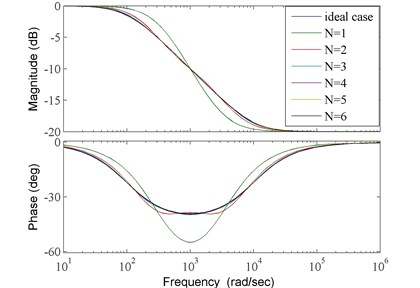The bigger approximation order $N$ is, the higher approximation accuracy is. But when $N$ reaches a certain value, approximation accuracy would not be proportionally increased . Take $\alpha =\text{0.5}$, ${w}_{b}=\text{100}$, ${w}_{h}={\text{10}}^{\text{4}}$ as a case study, the Bode plots of ideal case and its approximations with $N=$1, 2, 3, 4, 5, 6 are shown in Fig. 4. And we can find that big approximation error only exists in the approximation with $N=\text{1}$.

For further showing the differences in approximations with different orders, the mean absolute errors of frequency characteristics between ideal case and its approximations are employed as accuracy evaluation standards, they are defined as follows:

11
${E}_{mag}=\frac{1}{m+1}\sum _{i=0}^{m}|{M}_{E}\left({\omega }_{i}\right)|,$
12
${E}_{phase}=\frac{1}{m+1}\sum _{i=0}^{m}|{P}_{E}\left({\omega }_{i}\right)|,$
13
${M}_{E}\left({\omega }_{i}\right)=20{\mathrm{l}\mathrm{o}\mathrm{g}}_{10}|Q\left(j{\omega }_{i}\right)|-20{\mathrm{l}\mathrm{o}\mathrm{g}}_{10}|{G}_{N}\left(j{\omega }_{i}\right)|,$
14
${P}_{E}\left({\omega }_{i}\right)=\angle Q\left(j{\omega }_{i}\right)-\angle {G}_{N}\left(j{\omega }_{i}\right),$

where ${E}_{mag}$, ${E}_{phase}$ are the mean absolute errors of amplitude-frequency characteristic and phase-frequency characteristic respectively, ${w}_{0}={w}_{b}$, ${w}_{m}={w}_{h}$.

The evaluation results shown in Fig. 5 indicate that, the improvement of approximation accuracy is slight when approximation order is bigger than 4. Thus, 4th-order broken-line approximation for fractional order $Q$-filters would be adopted in this study.

Fig. 5Evaluation results of approximations with different orders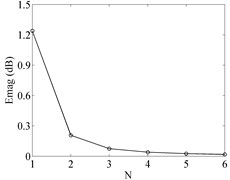a)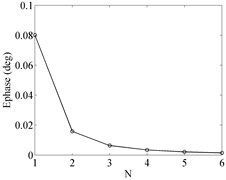b)

## 4. Numerical simulations

For showing the efficiency of the proposed control strategy, take an actual stabilized platform system which is driven by SER3112 AC synchronous servomotors as an example to analyze. Parameters of the studied stabilized platform system is shown in Table 1. With appropriate simplifications, the transfer function of control object in speed loop is:

15
${G}_{vob}\left(s\right)=\frac{R{G}_{pm}/{k}_{cf}}{{C}_{e}{T}_{m}s\left(2{T}_{\sum i}s+1\right)}=\frac{0.2261}{1.14844×{10}^{-6}{s}^{2}+3.1954×{10}^{-3}s},$

where ${C}_{e}$ is voltage constant, ${T}_{m}$ is mechanical time constant, $R$ is half of winding resistance ${R}_{U_V}$, ${G}_{pm}$ is the approximate proportional factor between platform rotational angular rate and motor speed, ${k}_{cf}$ is amplification of current feedback, and ${T}_{\sum i}={T}_{cf}+{T}_{PWM}$, ${T}_{PWM}$ and ${T}_{cf}$ are time constants of inverter and current feedback filter respectively.

Table 1Parameters of a stabilized platform system

 ${C}_{e}$ (V, value at 1000 rpm) ${T}_{m}$ (s) ${T}_{cf}$ (s) ${T}_{PWM}$ (s) ${R}_{U_V}$ (Ω) ${G}_{\text{pm}}$ ${k}_{\text{cf}}$ 140.0 $\text{2.39×}{\text{10}}^{\text{-3}}$ $\text{1.1×}{\text{10}}^{\text{-4}}$ $\text{6.97×}{\text{10}}^{\text{-5}}$ 18.1 $\text{1}/\text{60}$ 0.667

### 4.1. Example 1

First, examine system responses to a step signal while there is a disturbance $d=2+0.5\mathrm{s}\mathrm{i}\mathrm{n}\left(0.1\pi t\right)$ existing in system. Three kinds of controllers are used to regulate the system separately, they are proportional controller ${K}_{n}=\text{2}$, adaptive grey predictive controller (AGPC), and the newly proposed controller, fractional-order disturbance observer based adaptive grey predictive controller (FDOB-AGPC). The feedback regulators in AGPC and FDOB-AGPC both employ proportional controller ${K}_{n}=\text{2}$. And in grey prediction module, the number of prediction sequence is 4. Since grey prediction model has a relatively high accuracy for short-term forecasting, we take the maximum prediction step is 4. Via comparing, the dynamic prediction mode performs the big positive-step step ${\xi }_{3}=\text{4}$, small positive-step ${\xi }_{2}=\text{1}$ and negative-step ${\xi }_{1}=$–3 mode based on the tracking error $e$. Thus the switching mechanism is defined as follows:

16
$\xi =\left\{\begin{array}{ll}-3,& e>0.6\text{\hspace{0.17em}}r,\\ 1,& 0.1\text{\hspace{0.17em}}r\le e\le 0.6\text{\hspace{0.17em}}r,\\ 4,& e<0.1\text{\hspace{0.17em}}r.\end{array}\right\$

Eq. (15) indicates that fractional order $\alpha$ of $Q$-filter should be not less than 2, and to prevent the system from becoming too complex, we take $\alpha =\text{2.0,\hspace{0.17em}2.2,\hspace{0.17em}2.4,\hspace{0.17em}2.6,}\text{}\text{2.8,\hspace{0.17em}3.0}$ to study. Bode curves of $Q$-filter with different $\alpha$, $N=\text{4}$, and approximation bandwidth [100, 10000], are shown in Fig. 6. From Fig. 6 we can see that, changing the $Q$-filter’s order fractionally can effectively enlarge the range of control system’s frequency responses adjustment than just turning it among integer orders.

Fig. 6Bode curves of Q-filter with different α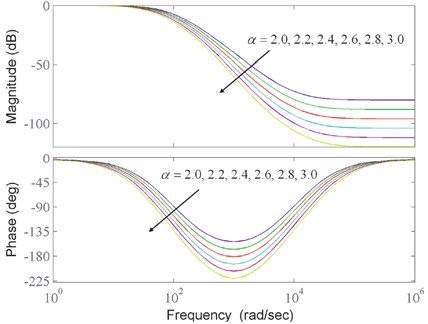Fig. 7System responses to the step signal with different controllers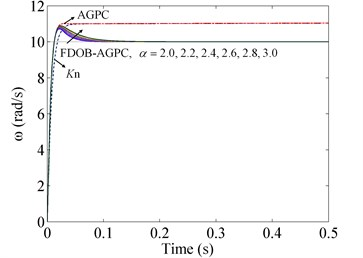a) Response curves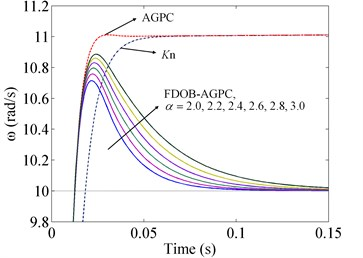b) Partial enlarged curves

System responses to the step signal with different controllers are depicted in Fig. 7. Via comparing the curve of AGPC with that of proportional controller, we can see that AGPC with adaptive steps can improve the response speed remarkably. But the two controllers both have steady-state errors because of the external disturbances, it means that they have poor suppression abilities for external disturbances. Whereas, the steady-state error no longer exists in the response curves of FDOB-AGPC, this implies FDOB-AGPC can give better vibration suppression performance with the help of FDOB. While the control system still keeps stable, the bigger fractional order $\alpha$ is, the more deteriorative the performance is. Since larger $\alpha$ gives more phase margin, simulation results verify a tradeoff between stability and the strength of vibration suppression exists. In addition, responses of FDOB-AGPC with different $\alpha$ indicate that fractional order can provide a wider adjustment range than integer order, and can make the adjustment alter continuously.

### 4.2. Example 2

To simulate the actual system truthfully, friction torque is used as the equivalent disturbance $d\text{,}$ and considering that plant ${G}_{p}\left(s\right)$ contains an multiplicative perturbation ${G}_{P}\left(s\right)={G}_{n}\left(s\right)+\mathrm{\Delta }P\left(s\right)$, $\mathrm{\Delta }P\left(s\right)=-0.1{G}_{n}\left(s\right)$. In this example, the aggregate friction model which is composed of static friction, Coulomb friction, viscous friction and Stribeck friction is employed. The friction moment can be defined as follows :

17
${M}_{f}=\delta \left(\stackrel{˙}{\theta }\right){M}_{static}+\left[1-\delta \left(\stackrel{˙}{\theta }\right)\right]{M}_{slide},$

where $\stackrel{˙}{\theta }$ is motor speed, $\delta \left(\stackrel{˙}{\theta }\right)$ is switching function, and ${M}_{static}$, ${M}_{slide}$ are static friction moment and sliding friction moment respectively:

18
${M}_{static}=\left\{\begin{array}{ll}{M}_{m},& M\left(t\right)\ge {M}_{m},\\ M\left(t\right),& -{M}_{m}
19
${M}_{slide}=\left[{M}_{c}+\left({M}_{m}-{M}_{c}\right){e}^{-{\alpha }_{1}\left|\stackrel{˙}{\theta }\right|}\right]\mathrm{s}\mathrm{g}\mathrm{n}\left(\stackrel{˙}{\theta }\right)+{k}_{v}\stackrel{˙}{\theta },$
20
$\delta \left(\stackrel{˙}{\theta }\right)=\left\{\begin{array}{cc}0,& \left|\stackrel{˙}{\theta }\right|>\alpha ,\\ 1,& \left|\stackrel{˙}{\theta }\right|\le \alpha ,\end{array}\right\$

where $M\left(t\right)=J\stackrel{¨}{\theta }$ is driving torque, ${M}_{m}$ is maximal static friction moment, ${M}_{c}$ is Coulomb friction moment, ${k}_{\text{v}}$ is proportional coefficient of viscous friction moment, ${\alpha }_{1}$ and $\alpha$ are both small constants. And in this study, there are ${M}_{m}=\text{0.6}\text{}\text{Nm}\text{,}$${M}_{\text{c}}=\text{0.5}\text{}\text{Nm}\text{,}$${\alpha }_{1}=\alpha =\text{0.01}\text{,}$${k}_{v}=\text{0.04}$.

Fig. 8Friction torque curves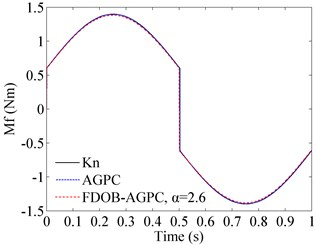Take sinusoidal signal $r=20\mathrm{s}\mathrm{i}\mathrm{n}\left(2\pi t\right)$ as reference input, and still employ the above three kinds of controllers to regulate the system. Except ${K}_{n}=\text{12}$ and $\alpha =\text{2.6}$, other configurations for these controllers are not changed. Friction torque curves are shown in Fig. 8, and system responses to the sinusoidal signal are depicted in Fig. 9.

Tracking curves in Fig. 9 illustrate that steady-state errors still exist when applying proportional controller and AGPC. As friction torques suddenly change at zero-crossing points of motor speed, mutations appear in the tracking processes as depicted in error curves. The sudden change of friction torque also has adverse influence on FDOB-AGPC, but FDOB-AGPC can adjust quickly, and its steady-state error is no more than 0.1 rad/s with the compensation action of FDOB. Thus, the results validate that when FDOB is introduced, the system has better robust stability.

Fig. 9Tracking abilities of sinusoidal signal with different controllers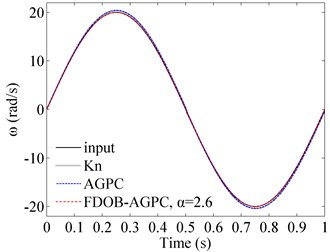a) Tracking curves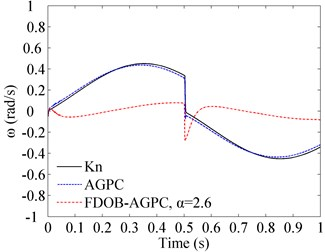b) Error curves

### 4.3. Example 3

For the same simulation conditions described in Example 2, we consider tracking a more complex signal to validate the effectiveness of the newly proposed controller. The reference input contains three processes, acceleration process, uniform process and rapid deceleration process. Tracking results of the three controllers are shown in Fig. 10.

Fig. 10Tracking abilities of a more complex signal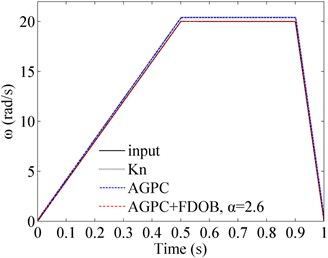a) Tracking curves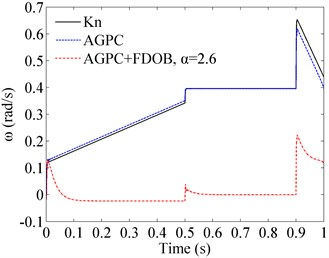b) Error curves

During the acceleration process, tracking errors of proportional controller and AGPC increase as the friction torques become bigger which are determined by the motor speed. From the uniform process we can see that, steady-state errors always exit when applying proportional controller and AGPC. And the relatively large deceleration brings worse impacts on the two controllers. The large deceleration also has adverse influence on FDOB-AGPC, but throughout the control process of FDOB-AGPC, big tracking errors mainly occur at the state transition points, and the biggest error is only 0.2229 rad/s. Comparing with proportional controller and AGPC, the overall control performance of FDOB-AGPC is encouraging. And the simulation results of this example is concordant with the ones of Example 2.

## 5. Conclusions

This paper introduces the design of a novel control strategy for vibration suppression, which combines adaptive grey predictive control and fractional-order disturbance observer. Numerical simulations are carried out by taking an actual stabilized platform system for case studies, and some conclusions are reached.

1) AGPC has a better performance of shortening the settling time while comparing with proportional controller.

2) The proposed control scheme FDOB-AGPC is shown superior to AGPC with respect to vibration suppression as compared.

3) Fractional-order disturbance observer has attractive flexibility than conventional disturbance observer as the order of $Q$-filter is expanded to real-number domain.

However, system output can be easily affected by external noise. And in Fig. 3, we know that DOB can make system characteristic approximately equal to the nominal model, but it is not good at suppressing high-frequency measurement noise . Hence, compared with conventional feedback controllers, the improving of measurement noise reduction ability of FDOB-AGPC is mainly depend on GM(1,1) model, which applies a preliminary transformation called accumulated generating operation to reduce the noise. And in order to improve the measurement noise reduction ability ulteriorly, the author’s previous work which uses an additional control signal to compensate system output $y$ in DOB  can be referred to.